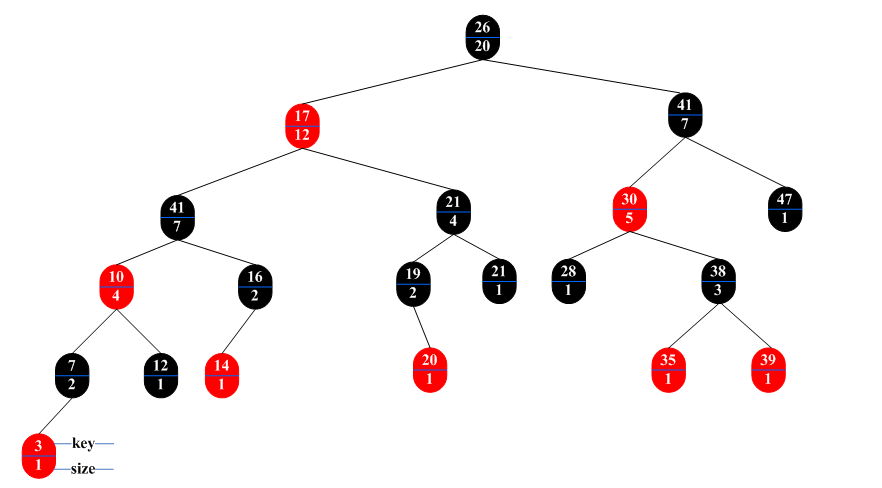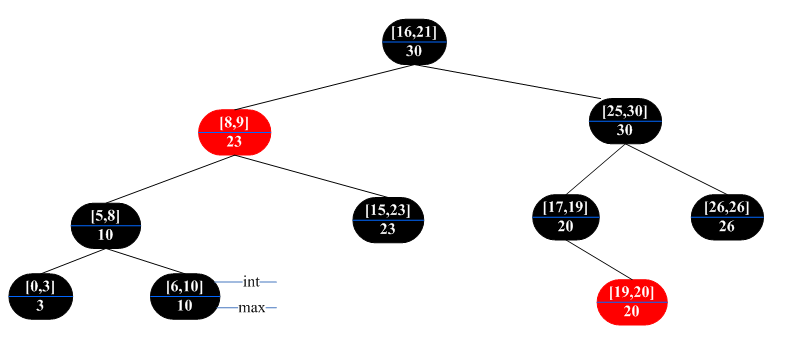2014-06-19 11:20:40 loveprogram_1 阅读数 994
• ###### iOS开发教程之OC语言

Objective-C是扩充C的面向对象编程语言，iOS开发是用的Objective-C语言，本专题更系统的讲解Objective-C语言当中的一些要点，类的封装、基本数据结构(NSString、NSData)、继承、内存管理(retain点语法、MyArray、AutoreleasePool、浅拷贝详述、深拷贝详述)等内容。

40600 人正在学习 去看看 欧阳坚

为应对新的应用需求，经常是通过存储额外信息的方法来扩张一种标准的数据结构，

【已知】对于一个无序的集合，能够在O(n)的时间内确定任何的顺序统计量。
rank(x)：获得x在数组中的位置，遍历数组并统计比x.key小的元素数即可。
select(i)：利用random-partion()函数，二分地去定位即可。
【目标】修改红黑树，使得在O(lgn)时间内确定任何的顺序统计量。
【顺序统计树】在每个结点上存储附加信息的一棵红黑树。

```OS-SELECT( x, i ) // 过程返回一个指针，指向以x为根的子树中包含第i小关键字的结点
r = x.left.size + 1
if i == r
return x
else if i < r
return OS-SELECT( x.left, i )
else
return OS-SELECT( x.right, i - r )
OS-RANK( T, x ) // 过程返回T中序遍历对象的线性序中x的位置
r = x.left.size + 1
y = x
while y != T.root
if y == y.p.right
r += y.p.left.size + 1
y = y.p
return r```
【OS-RANK解释】

【对子树规模的维护】
LEFT-ROTATE( T, x )的代码，要添加下面两行：
y.size = x.size // y = x.right
x.size = x.left.size + x.right.size + 1
RIGHT-ROTATE( T, x )的代码，要添加下面两行：
y.size = x.size
x.size = x.left.size + x.right.size + 1

（1）选择一种基础数据结构。（red-black tree）
（2）确定基础数据结构中要维护的附加信息。(subtree size,直接记录秩也可以，不过速度太慢了)
（3）检验基础数据结构上的基本修改操作能否维护附加信息。（Insert、Delete/Rotate是否可以维持子树大小的信息）

（4）设计一些新操作。（怎么使用这些信息：OS-Select、OS-Rank）

【实际使用时】上面可能更像一个checklist，而设计时可以先设计一个些新操作（4），再去验证附加信息维护等。

【2+2】2组数据 + 2组操作：基础操作+附加操作 ： 基本操作+新添操作

【区间i与i'重叠(overlap)】

（1）i和i'重叠。
（2）i在i'的左边，即i.high < i'.low

（3）i在i'的右边，即i.low > i'.high

Example Interval Trees ： Maintain a set of intervals.

eg : time intervals.

【区间树】一种对动态集合进行维护的红黑树，其中每个元素x都包含一个区间x.int。

（1）INTERVAL-INSERT( T, x )：将包含区间属性的int的元素x插入到树T中。
（2）INTERVAL-DELETE( T, x )：从T删除x。
（3）INTERVAL-SEARCH( T, i )：返回一个指向T中元素x的指针，使x.int与i重叠。不存在返回T.nil。
【扩张数据结构】

```INTERVAL-SEARCH( T, i )
x = T.root
while x != T.nil and i does not overlap x.int
if x.left != T.nil and x.left.max >= i.low // i'.low <= i.high
x = x.left
else
x = x.right
return x```
【解释】

i'.high = x.left.max >= i.low，

========================================================================

【图示化补充】

【顺序统计树】下面给出一个修改后的红黑树的例子，如下图所示：【区间树】修改红黑树得到的区间树如下图所示：2019-05-22 09:49:49 weixin_40205234 阅读数 266
• ###### iOS开发教程之OC语言

Objective-C是扩充C的面向对象编程语言，iOS开发是用的Objective-C语言，本专题更系统的讲解Objective-C语言当中的一些要点，类的封装、基本数据结构(NSString、NSData)、继承、内存管理(retain点语法、MyArray、AutoreleasePool、浅拷贝详述、深拷贝详述)等内容。

40600 人正在学习 去看看 欧阳坚

### 一、动态顺序统计

一种支持一般动态集合上顺序统计操作的数据结构。通过这种数据结构，可以快速找到一个集合中的第i小的数，（select）或给出一个指定元素在集合的全序中的位置。（rank）

【思想】添加新项：在红黑树的结点上记录下该结点的子树个数。size[x] = size[left[x]] + size[right[x]] +1。 若结点为空，则为0。

``````BSTNode* OSRBTree::os_select(BSTNode *p, const int &ith){
if(p == NULL) return p;
int k = 1;
if(p->left != NULL){
k = p->left->size + 1;           // 当前该结点所对应的rank

}
if(ith == k) return p;
if(ith < k) return os_select(p->left, ith);
else return os_select(p->right, ith - k);
}
``````

``````//  return the rank of value
int OSRBTree::os_rank(BSTNode *p, const int &value){
if(p == NULL) return 0;
int k = 1;
if(p->left != NULL)
k = p->left->size + 1;
if(p->val == value)
return k;
else if(p->val > value) return os_rank(p->left, value);
else return os_rank(p->right, value)+k;
}
``````

1：选择一个基础的数据结构(red-black tree)

2：在数据统计中维护一些附加信息(子树大小)

3：验证这个数据结构上的信息不会受修改操作的影响(insert, delete---rotations)

4：建立新的运算。假设新的数据已经存好了，然后开始使用这些信息(os_select, os_rank).

### 二、区间树(Interval Tree)

【图示化补充】

1：选择红黑树作为基本的数据结构，并将区间的较低值(low)作为键值

2：将结点子树的最大值作为新添加的项(m[x] = max{high[int[x]],m[left[x]], m[right[x]]}).

3：是否受插入删除等操作的影响？受，但是在O(1)时间内就能调整过来，见代码。

4：新的操作，查询集合中与给定区间重叠的一个区间。

``````#ifndef INTERVALTREE
#define INTERVALTREE
#include <iostream>
#include <string>
using namespace std;
struct dataNode{
int low;
int high;
};
class BSTNode{
public:
BSTNode *left, *right;
BSTNode *parent;
int val;
dataNode d;
string color;
int m;         // 最大值
};

class IntervalTree{
public:
IntervalTree(const dataNode &d)
{
root = new BSTNode();
root->d = d;
root->color = "black";
root->left = NULL;
root->right = NULL;
root->m = d.high;
root->parent = NULL;
root->val = d.low;
}

BSTNode* insertBST(BSTNode *p, const dataNode &d);
void insertIntervalTree(BSTNode *root1, const dataNode &d);
void inorderOSRBTree(BSTNode *p);
BSTNode* intervalSearch(BSTNode *p, const dataNode &d);
public:
BSTNode *root;
void destroyBST(BSTNode *p);
};

#endif``````

### 【顺序统计树】下面给出一个修改后的红黑树的例子，如下图所示：### 【区间树】修改红黑树得到的区间树如下图所示：2017-11-04 10:52:05 u010385790 阅读数 151
• ###### iOS开发教程之OC语言

Objective-C是扩充C的面向对象编程语言，iOS开发是用的Objective-C语言，本专题更系统的讲解Objective-C语言当中的一些要点，类的封装、基本数据结构(NSString、NSData)、继承、内存管理(retain点语法、MyArray、AutoreleasePool、浅拷贝详述、深拷贝详述)等内容。

40600 人正在学习 去看看 欧阳坚

1.    动态有序统计：（SELECT在动态集中返回第i小的数，RANK在有序集中返回排名为i的元素）

【已知】对于一个无序的集合，能够在O(n)的时间内确定任何的顺序统计量

【目标】修改红黑树，使得在O(lgn)时间内确定任何的顺序统计量

——>顺序统计树: 在每个结点上存储附加信息的一棵红黑树。

【节点信息】附加属性x.size = x.left.size + x.right.size + 1

【对子树规模的维护】维护size时间复杂度：O(1)。所以插入、删除，包括维护size属性，都只需要O(lgn)时间。

OS-SELECT( x, i ) // 过程返回一个指针，指向以x为根的子树中包含第i小关键字的结点

r= x.left.size + 1

if i == r

return x

else if i < r

return OS-SELECT( x.left, i )

else

return OS-SELECT( x.right, i - r )

OS-RANK( T, x ) // 过程返回T中序遍历对象的线性序中x的位置

r= x.left.size + 1

y= x

while y != T.root

if y == y.p.right

r += y.p.left.size + 1

y = y.p

return r2.    区间树（找到与目标区间重合的区间）

【节点信息】每个结点的关键字为区间int（低端点x.int.low）。附加信息包含一个值x.max，它是以x为根的子树中，所有区间的端点的最大值。

【对子树规模的维护】一次旋转后，更新max属性只要O(1)的时间；所以插入和删除的运行时间为O(lgn)http://blog.csdn.net/loveprogram_1/article/details/32318211

http://blog.csdn.net/lu597203933/article/details/43459035

2015-04-26 23:28:33 lth404391139 阅读数 894
• ###### iOS开发教程之OC语言

Objective-C是扩充C的面向对象编程语言，iOS开发是用的Objective-C语言，本专题更系统的讲解Objective-C语言当中的一些要点，类的封装、基本数据结构(NSString、NSData)、继承、内存管理(retain点语法、MyArray、AutoreleasePool、浅拷贝详述、深拷贝详述)等内容。

40600 人正在学习 去看看 欧阳坚

1.选择一个基本的数据结构 例如红黑树
2.决定要添加到结点的基本信息  例如实现查询第k大数功能，应添加的基本信息为所有子树结点之和，而非直接保存该结点键值的秩
3 维持 插入+旋转/删除+旋转

4 封装为函数，实现其功能

2015-07-21 11:03:43 sinat_28626091 阅读数 379
• ###### iOS开发教程之OC语言

Objective-C是扩充C的面向对象编程语言，iOS开发是用的Objective-C语言，本专题更系统的讲解Objective-C语言当中的一些要点，类的封装、基本数据结构(NSString、NSData)、继承、内存管理(retain点语法、MyArray、AutoreleasePool、浅拷贝详述、深拷贝详述)等内容。

40600 人正在学习 去看看 欧阳坚

（1）以红黑树作为underlying data structure，在树的每个节点增加一个int域储存该节点的subtree节点个数（包含自身）

node_size(x) = node_size(left[x]) + node_size(right[x]) + 1;

```typedef struct node{
int key;    int color;  //Augment field: the number of all nodes in the subtree of current node, including its self
struct node *left;  struct node *right;  struct node *p;
int node_size();
}*pnode, node;
node sentinel = {0,1,NULL,NULL,NULL};  //初始化列表赋值，initializer_list
pnode nil= &sentinel;

int node::node_size(){
if(this != nil)
return left->node_size()+right->node_size()+1;
else
return 0;
}```

```void test_RBtree(){
vector<int> A = {7,3,18,10,22,8,11,26};
pnode root = nil;
for(int i = 0; i < A.size(); i++){
pnode z = (pnode)malloc(sizeof(node));
z->key = A[i];  z->left = nil;  z->right = nil;
RB_insert(root, z);
}

inorder_walk(root);
cout<<endl;

pnode z = (pnode)malloc(sizeof(node));   z->left = nil;  z->right = nil;  z->key = 15;
RB_insert(root, z);
pnode rr = find_root(z);

inorder_walk(rr);
cout<<endl;
cout<<rr->node_size()<<endl;
}```（2）order statistic：OS_select()以及OS_rank:

```int OS_rank(pnode x){
int r = x->right->node_size();
while(x == x->p->right)
x = x->p;
return x->node_size()-r;
}

pnode OS_select(pnode root, int rank){
if(root->node_size() >= rank){
int r = root->right->node_size();
if(root->node_size()-r == rank)
return root;
if(root->node_size()-r > rank)
return OS_select(root->left, rank);
if(root->node_size()-r < rank)
return OS_select(root->right, rank-root->node_size()+r);
}
return nil; //如果给出的rank值超出树的大小，则返回nil指针
}```

（3）Update subtree sizes when inserting or deleting, 与第（1）种方法不同，在node内直接定义一个int size域，对RB_insert进行修改，使其在插入时就直接修改各个相关节点的size大小。注意rotation操作需要修改size大小，时间复杂度为O(1)：just look at children size. （待完成